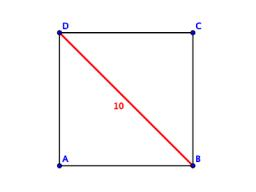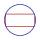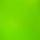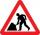# Square

Calculate the side of a square with a diagonal measurement 10 cm.

x =  7.0711 cm

### Step-by-step explanation:Did you find an error or inaccuracy? Feel free to write us. Thank you!Tips to related online calculators
Pythagorean theorem is the base for the right triangle calculator.

#### You need to know the following knowledge to solve this word math problem:

We encourage you to watch this tutorial video on this math problem:

## Related math problems and questions:

• Two diagonalsThe rhombus has a side length of 12 cm and a length of one diagonal of 21 cm. What is the length of the second diagonal?
• Diagonal of squareCalculate the side of a square when its diagonal is 10 cm.
• RectangleThe rectangle is 18 cm long and 10 cm wide. Determine the diameter of the circle circumscribed to the rectangle.
• CuboidCuboid with edge a=6 cm and space diagonal u=31 cm has volume V=900 cm3. Calculate the length of the other edges.
• Diagonal of the rectangleCalculate the rectangle's diagonal, which area is 54 centimeters square, and the circuit is equal to 30 cm.
• Square2Side of the square is a = 6.2 cm, how long is its diagonal?
• Body diagonalThe cuboid has a volume of 32 cm3. Its side surface area is double as one of the square bases. What is the length of the body diagonal?
• Rhombus and inscribed circleIt is given a rhombus with side a = 6 cm and the radius of the inscribed circle r = 2 cm. Calculate the length of its two diagonals.
• Chord ABWhat is the chord AB's length if its distance from the center S of the circle k(S, 92 cm) is 10 cm?
• Square s3Calculate the diagonal of the square, where its area is 0.49 cm square. And also calculate its circumference.
• Square diagonalCalculate the length of the square diagonal if the perimeter is 172 cm.
• DiagonalsA diagonal of a rhombus is 20 cm long. If it's one side is 26 cm, find the length of the other diagonal.
• DiagonalCalculate the diagonal length of the rectangle ABCD with sides a = 8 cm, b = 7 cm.
• HypotenuseCalculate the length of the hypotenuse of a right triangle with a catheti 71 cm and 49 cm long.
• A rectangle 2A rectangle has a diagonal length of 74cm. Its side lengths are in ratio 5:3. Find its side lengths.
• Square diagonalCalculate the length of diagonal of the square with side a = 11 cm.
• Diagonal - simpleCalculate the length of the diagonal of a rectangle with dimensions 5 cm and 12 cm.